# RedditDataScience

## CMSC320 Final Project

AnalyzeReddit# Rock The Upvote:

## Predicting the Success of a Reddit Post

Neehar Peri, Wilson Orlando

## Introduction

Ever heard of Reddit? Maybe you already have; it's the 6th most visited website in the U.S. If this is your first time hearing about it, Reddit is a "social news aggregation forum", which is the official way of saying it gathers content from all over the internet to display in one place. Users submit content to Reddit like links, text posts, and images, and that content gets liked ("upvoted") or disliked ("downvoted") by other users. Posts are grouped into user-created boards called "subreddits" focused on one topic- like cute animals, world news, or Game of Thrones- and the most upvoted posts across all subreddits are shown on the site's front page. You can read more about it here.

With 330 million users and over 20 thousand daily submissions, it’s hard to predict which posts will get popular enough to hit the front page. If your predictions were good enough to craft viral Reddit posts, though, you could get your ideas out to those millions of users in a matter of hours. Many users don’t realize that Reddit can be- and is already being- manipulated to spread more than cute cats. Large financial service companies use Reddit to boost their online image, and just this month the Reddit team claimed leaked U.S.-U.K. trade documents posted on their site were part of a large-scale misinformation campaign originating from Russia. On top of getting your own upvotes, understanding what makes a Reddit post popular is critical for your informed online browsing.

Given the huge amount of data Reddit generates, it’s also an interesting problem for data science: based on existing Reddit posts, can we identify the most important features of a successful post and predict a post’s success before it’s submitted? In this tutorial, our goal is to download and tidy data from Reddit, perform some exploratory analysis to identify important features, then use machine learning models to predict a post’s upvote rating based on those features. For readers unfamiliar with Reddit, we hope our analysis will get you interested in the site and in some popular posts. For more experienced readers, we hope to give you some insight into how your favorite “social news aggregation forum” works, and show you how to get your own posts to the front page of the internet.

## Getting Started with the Data

We use Python 3 and some imported libraries, like torch, pandas, matplotlib, scikit-learn, and more.

In :
from textblob import TextBlob
import torch
import torch.nn as nn
import torch.optim as optim
import pandas as pd
import matplotlib.pyplot as plt
import seaborn as sns
from sklearn.linear_model import Lasso, LogisticRegression
import sklearn.metrics as metrics
from sklearn.model_selection import train_test_split
from tqdm import tqdm
from pprint import pprint
import numpy as np
import praw
import pdb
import operator
import warnings
warnings.filterwarnings("ignore")


### Data Collection

To scrape data from Reddit, we'll be using PRAW (the Python Reddit API Wrapper). For this analysis, we chose to pull data into two datasets:

1. Top Posts: The 1000 most upvoted posts in the last year
2. Controversial Posts: The 1000 most controversial posts in the last year (i.e. closest to 50% upvote ratio)
This gives us a good spread of recent posts that have been wildly successful and unsuccessful.

In :
# Establish connection using Reddit's API
clientID = 'VpVEXRwC5-nrbA'
clientSecret = 'XMql3JHOqhq2NatetFMFPkhBaCE'
userAgent = 'Reddit WebScraping'
reddit = praw.Reddit(client_id=clientID, client_secret=clientSecret, user_agent=userAgent)

In [ ]:
# Retrieve the top (most upvoted) 1k posts in the last year and add them to a Pandas dataframe
topPosts = []
subReddit = reddit.subreddit('all')
for post in tqdm(subReddit.top(time_filter = 'year', limit=1000), total=1000):
topPosts.append([post.title, post.score, post.id, post.subreddit, post.url, post.num_comments, post.selftext, post.created_utc, post.author, post.is_self, post.over_18, post.spoiler, post.upvote_ratio])
topPosts = pd.DataFrame(topPosts,columns=['title', 'score', 'id', 'subreddit', 'url', 'num_comments', 'body', 'created', 'author', 'is_self', 'over_18', 'spoiler', 'upvote_ratio'])
topPosts.to_csv("top1KPosts.csv", index=False)

# Retrieve the most controversial (most downvoted) 1k posts in the last year and add them to a Pandas dataframe
controvertialPosts = []
subReddit = reddit.subreddit('all')
for post in tqdm(subReddit.controversial(time_filter = 'year', limit=1000), total=1000):
controvertialPosts.append([post.title, post.score, post.id, post.subreddit, post.url, post.num_comments, post.selftext, post.created_utc, post.author, post.is_self, post.over_18, post.spoiler, post.upvote_ratio])
controvertialPosts = pd.DataFrame(controvertialPosts,columns=['title', 'score', 'id', 'subreddit', 'url', 'num_comments', 'body', 'created', 'author', 'is_self', 'over_18', 'spoiler', 'upvote_ratio'])
controvertialPosts.to_csv("controvertial1KPosts.csv", index=False)

In :
top1KPosts = pd.read_csv("top1kPosts.csv")


To avoid calling the Reddit API thousands of times, we have saved the results of 1000 "Top" posts and 1000 "Controversial" posts to a Pandas dataframe. For all future analysis, we will be reading and modifying these data frames.

Next, let's take a look at some of the subreddits that have broken the top 1000 and controversial 1000 posts:

In :
#Top 10 List of subreddits that have broken top 1k
topSubReddit = top1KPosts.subreddit.unique()
topSubReddit[0:10]

Out:
array(['pics', 'gaming', 'AskReddit', 'Showerthoughts', 'news', 'memes',
'funny', 'YouFellForItFool', 'aww', 'videos'], dtype=object)
In :
 #Top 10 subreddits that have broken most controversial 1k
controversialSubReddits = controversial1KPosts.subreddit.unique()
controversialSubReddits[0:10]

Out:
array(['motorcycles', 'unpopularopinion', 'IAmA', 'AmItheAsshole',
'DestinyTheGame', 'FortNiteBR'], dtype=object)

Our sampling of subreddits in the top 1000 are pretty predictable; r/pics, r/aww, and r/AskReddit are some of the biggest subreddits on the site ("r/" denotes a subreddit's name), with AskReddit hosting over 25 million subscribed users. This would indicate that bigger subreddits are more likely to break the top 1000, so a post's subreddit should be a very important feature for its success.

The controversial sample of subreddits shows a few different trends. Of the 74 subreddits that have broken the controversial 1000, many (like r/unpopularopinion and r/TrueOffMyChest), are communities for people to vent intentionally controversial ideas to other people. There's also a sizeable chunk of political subreddits, like r/Libertarian and r/Conservative, which also host a lot of controversial opinions (as is the nature of partisan politics). Surprisingly, though, the vast majority of controversial subreddits have to do with popular entertainment, like r/DestinyTheGame, r/leagueoflegends, and r/FortNiteBR. Part of this is sampling bias; there's inherently just a lot of subreddits dedicated to popular entertainment on Reddit, so it makes sense that they'd make up a significant percentage of the controversial dataset. Interestingly, though, many of them reflect legitimate controversies in the past year! r/gameofthrones is present, after many fans were disappointed with the show's final season, and r/Blizzard makes an appearance after the gaming company punished prominent players for supporting the Hong Kong riots.

For our analysis, then, we'd recommend posting to large generic subreddits and avoiding specific potentially-controversial ones, hypothesizing that a post's subreddit is an important feature in its success.

### Data Preprocessing

Next, we'll have to clean up the data from PRAW to get it into a more usable format. We'll be combining the top and controversial posts into a single Pandas dataframe, adding a field to differentiate between them, and dropping some fields that could not be controlled by a user at the time of post submission (like url and reddit's internal post id).

In :
for tableRow in top1KPosts.iterrows():
top1KPosts.at[tableRow, "post_type"] = 1 #Set topPost as post_type 1

for tableRow in controversial1KPosts.iterrows():
controversial1KPosts.at[tableRow, "post_type"] = 0 #Set controversialPost as post_type 0

dataSet = pd.concat([top1KPosts, controversial1KPosts])
dataSet = dataSet.reset_index()
dataSet = dataSet.drop(["index", "id", "url", "created", "author"], axis=1) #Drop Features that cannot be controlled by the user

Out:
title score subreddit num_comments body is_self over_18 spoiler upvote_ratio post_type
0 Given that reddit just took a $150 million inv... 228918 pics 6491 NaN False False False 0.94 1.0 1 I got off the horse by accident right before a... 226498 gaming 2238 NaN False False False 0.97 1.0 2 Take your time, you got this 224055 gaming 3360 NaN False False False 0.97 1.0 3 People who haven't pooped in 2019 yet, why are... 221862 AskReddit 8132 NaN True False False 0.91 1.0 4 Whoever created the tradition of not seeing th... 218614 Showerthoughts 2098 Damn... this got big... True False False 0.96 1.0 From here, we'll conduct all analysis on this master dataset. Although metrics such as number of comments, post type, and upvote ratio cannot be controlled at the time of submission, we'll be keeping them as potential target metrics for later classification and regression. We still need to perform some data cleaning to make these features useable in machine learning models: In : uniqueSubReddits = {"subReddit" : []} #Get unique subReddits. for tableRow in dataSet.iterrows(): #Iterate through all rows in data set. title = tableRow["title"] subReddit = tableRow["subreddit"] body = tableRow["body"] originalContent = tableRow["is_self"] nsfw = tableRow["over_18"] spoiler = tableRow["spoiler"] titleBlob = TextBlob(title) lenTitle = len(title) titleSentiment = titleBlob.sentiment.polarity #Sentiment score from [-1, 1] -1 -> Negative, 1-> Positive titleSubjectivity = titleBlob.sentiment.subjectivity #Opinion score from [0, 1] 0 -> Factual, 1 -> Opinion titleQuestion = 1 if "?" in title else 0 #Is the title a question? if subReddit not in uniqueSubReddits["subReddit"]: uniqueSubReddits["subReddit"].append(subReddit) lenBody = 0 try: if np.isnan(body): #Setting empty body elements to empty strings to homogenize the data type within the column body = "" lenBody = 0 except: # Body is not NAN (Throws Exception) lenBody = len(body) #Set cleaned values and additional features dataSet.at[tableRow, "len_title"] = lenTitle dataSet.at[tableRow, "title_question"] = titleQuestion dataSet.at[tableRow, "title_sentiment"] = titleSentiment dataSet.at[tableRow, "title_subjectivity"] = titleSubjectivity dataSet.at[tableRow, "body"] = body dataSet.at[tableRow, "len_body"] = lenBody #Convert True -> 1 and False -> 0 dataSet.at[tableRow, "is_oc"] = 1 if originalContent else 0 dataSet.at[tableRow, "is_nsfw"] = 1 if nsfw else 0 dataSet.at[tableRow, "is_spoiler"] = 1 if spoiler else 0 dataSet = dataSet.drop(["is_self", "over_18", "spoiler",], axis=1) subRedditLookUp = pd.DataFrame(uniqueSubReddits) #Create table to iterate through all sub-reddits subRedditOneHotEncoding = pd.get_dummies(subRedditLookUp) #One hot encoding of categorical feature for input into model dataSet.head()  Out: title score subreddit num_comments body upvote_ratio post_type len_title title_question title_sentiment title_subjectivity len_body is_oc is_nsfw is_spoiler 0 Given that reddit just took a$150 million inv... 228918 pics 6491 0.94 1.0 241.0 0.0 0.245455 0.484848 0.0 0.0 0.0 0.0
1 I got off the horse by accident right before a... 226498 gaming 2238 0.97 1.0 67.0 0.0 0.028571 0.311905 0.0 0.0 0.0 0.0
2 Take your time, you got this 224055 gaming 3360 0.97 1.0 28.0 0.0 0.000000 0.000000 0.0 0.0 0.0 0.0
3 People who haven't pooped in 2019 yet, why are... 221862 AskReddit 8132 0.91 1.0 87.0 1.0 -0.100000 0.433333 0.0 1.0 0.0 0.0
4 Whoever created the tradition of not seeing th... 218614 Showerthoughts 2098 Damn... this got big... 0.96 1.0 189.0 0.0 0.000000 0.500000 23.0 1.0 0.0 0.0

The Reddit API makes data cleaning fairly straightforward. There are very few missing values, and all elements in the table are uniformly formatted, making it easier to specify rules to clean them. In addition to the features we are given through the API, we decided to calcualte a few other metrics that might be useful in determining the quality of a post. Specifically, we also look at the sentiment of the tilte, the objectivity of the title, length of the title, and length of the body. For the sake of simplicity, we do not consider the raw text data in a post to avoid dealing with links, subreddit specific acronyms and internet slang in general. Using these hand-crafted features, we will attempt to both regress the upvote ratio, and classify whether a post is considered a top post or a controversial post. Note that we choose to regress the upvote ratio rather than the total score because the score contains a very large range of values, making it difficult to accurately regress the values.

Now that our cleaning process is complete, it may help to define exactly what each of our features represents:

• title: The title of the post
• subreddit: The subreddit this post was made on
• body: The text written in the post's body, if any
• post_type: Whether this post came from our top (1) or controversial (0) dataset.
• len_title: The number of characters in the post's title
• title_question: Whether or not the post's title contains the '?' character.
• title_sentiment and title_subjectivity: Sentiment and subjectivity ratings of the title, as given by TextBlob (https://textblob.readthedocs.io/en/dev/quickstart.html#sentiment-analysis).
• len_body: Number of characters in the post's body text
• is_oc: 1 if the post is an original text post, 0 otherwise (i.e. it links to an external website with no body text)
• is_nsfw: 1 if the post is "nsfw" (inappropriate for some audiences), 0 otherwise
• is_spoiler: 1 if the post is tagged as a spoiler, 0 otherwise

## Exploratory Data Analysis

Now that our data has been assembled, we'd like to take a closer look at the kinds of subreddits in each category. If you're making a post, what kind of subreddit should you post on to ensure its success?

To answer this question, let's take a look at the subreddits that most frequently broke the top 1000 posts in the last year. The following plot shows the subreddits which had at least 10 posts in the top 1000 in the last year:

In :
# Plot subreddits that most frequently broke top 1000
subs = {}
for tableRow in dataSet.iterrows():
# Tally how many times each subreddit appears in dataset
if (tableRow["post_type"] == 1): # Filter only top posts
subreddit = tableRow["subreddit"]
if subreddit in subs:
subs[subreddit] = subs[subreddit] + 1
else:
subs[subreddit] = 1
top_subs = subs

# Filter out subs that broke top 1000 less than 10 times
consistent_subs = {}
for sub in subs:
if subs[sub] >= 10:
consistent_subs[sub] = subs[sub]

# Create bar chart
plt.bar(range(len(consistent_subs)), list(consistent_subs.values()), align='center')
plt.xticks(range(len(consistent_subs)), list(consistent_subs.keys()), rotation='vertical')
plt.title("Most Frequent Subreddits in Top 1000")
plt.ylabel("Number of Posts")
plt.xlabel("Subreddit Name")
plt.show()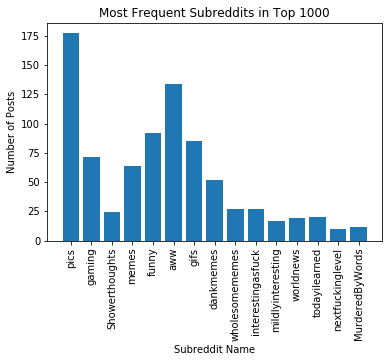As expected, all of these are large, popular subreddits. All of them (except r/Showerthoughts) primarily post links to external sites, like images, gifs, or videos. More notably, all of them have broad, generic themes, implying our previous hypothesis that more specific subreddits were more controversial had some merit. Next, then, let's plot the subreddits that broke the most controversial 1000 at least 10 times:

In :
# Plot subreddits that most frequently broke controversial 1000
subs = {}
for tableRow in dataSet.iterrows():
# Tally how many times each subreddit appears in dataset
if (tableRow["post_type"] == 0): # Filter only controversial posts
subreddit = tableRow["subreddit"]
if subreddit in subs:
subs[subreddit] = subs[subreddit] + 1
else:
subs[subreddit] = 1
cont_subs = subs

# Filter out subs that broke controversial 1000 less than 10 times
consistent_subs = {}
for sub in subs:
if subs[sub] >= 10:
consistent_subs[sub] = subs[sub]

# Create bar chart
plt.bar(range(len(consistent_subs)), list(consistent_subs.values()), align='center')
plt.xticks(range(len(consistent_subs)), list(consistent_subs.keys()), rotation='vertical')
plt.title("Most Frequent Subreddits in Controversial 1000")
plt.ylabel("Number of Posts")
plt.xlabel("Subreddit Name")
plt.show()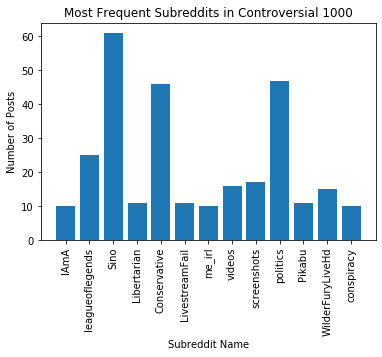As expected, there's a heavy presence of political subreddits like r/Sino, r/politics, r/Conservative, and r/Libertarian, as well as a popular entertainment subreddit (r/leagueoflegends). Unfortunately, it looks like there's some overlap with our top 1000 subreddits; r/IAmA and r/videos are popular subreddits, so subscriber count may be a poor predictor. As a follow-up, let's try this: what percentage of subreddits appear in either the top 1000 or the controversial 1000, but not both?

In :
# Count how many subreddits appear in either top or controversial, not both
unique_subs = {}
subs_count = len(top_subs)

for key in top_subs:
if key not in cont_subs:
unique_subs[key] = top_subs[key]

for key in cont_subs:
if key not in top_subs:
subs_count = subs_count + 1
unique_subs[key] = cont_subs[key]

len(unique_subs)/subs_count

Out:
0.9246376811594202

Aha! This is good; 92% of our subreddits are unique to either the top or controversial lists, so only 8% of subreddits appear on both. Even though subscriber count isn't a good metric, then, it looks like subreddit still is! We'll expect our machine learning models to count subreddit as an important feature, and they should perform decently well.

## Hot or Not?

From here, we'd like to put our hypotheses to the test. Are our features good enough to predict a post's success at the time of submission?

### Linear Regression

First, we're going to shoot the moon; let's try predicting the exact upvote ratio that a post will achieve based on its initial conditions. This is a regression problem, so let's try fitting our data to a linear regression model with "upvote_ratio" as our labels.

In :
inputRegressionData = []
outputRegressionData = []

#Creating examples with all features and the corresponding label
for tableRow in dataSet.iterrows():
subReddit = tableRow["subreddit"]
SR_oneHotEncoding = subRedditOneHotEncoding["subReddit_" + subReddit].to_list()
originalContent = tableRow["is_oc"]
nsfw = tableRow["is_nsfw"]
spoiler = tableRow["is_spoiler"]
lenTitle = tableRow["len_title"]
titleQuestion = tableRow["title_question"]
titleSentiment = tableRow["title_sentiment"]
titleSubjectivity = tableRow["title_subjectivity"]
lenBody = tableRow["len_body"]
ratio = tableRow["upvote_ratio"]

inputRegressionData.append(SR_oneHotEncoding + [titleQuestion, lenTitle, titleSentiment, titleSubjectivity, lenBody, originalContent, nsfw, spoiler])
outputRegressionData.append([ratio])


We use a Lasso model to build a linear model of the data since the traditional least squares method does not work well on sparse data.

In :
trainInput, testInput, trainOutput, testOutput = train_test_split(inputRegressionData, outputRegressionData, test_size=0.1) #Split data
ratioRegression = Lasso().fit(np.array(trainInput), np.array(trainOutput)) # Works better with sparse data
predictedOutput = ratioRegression.predict(np.array(testInput)) #Test the fitted model against unseen data

modelPerformance = []

testOutput = [i for i in testOutput]
predictedOutput = predictedOutput.tolist()
for i, output in enumerate(zip(testOutput, predictedOutput)):
target, prediction = output
modelPerformance.append({"Example" : i, "True Ratio" : target , "Predicted Ratio" : prediction, "Residual" : target - prediction})

modelPerformance = pd.DataFrame(modelPerformance)
MSE = metrics.mean_squared_error(testOutput, predictedOutput) #How close are the predictions to the actual values?
averageResidual = np.mean(modelPerformance["Residual"]) #Gives an idea if the model is consistently under predicting or overpredicting, indicating a poor fit


Out:
Example True Ratio Predicted Ratio Residual
0 0 0.50 0.760761 -0.260761
1 1 0.51 0.668199 -0.158199
2 2 0.50 0.703810 -0.203810
3 3 0.47 0.760761 -0.290761
4 4 0.91 0.760761 0.149239
In :
print("Mean Squared Error: " + str(MSE))
print("Average Residual: " + str(averageResidual))

Mean Squared Error: 0.042469174299435246
Average Residual: -0.04101170171391546


Directly regressing the ratio is extremely difficult since almost all ratios on reddit are between 0.5 and 1. In general, the linear model is able to minimize the loss function by predicting around 0.75 for each example. So, it looks like our data isn't fit well by a standard linear regression.

Given that this regression model performed badly, we'd like to pursue two different solutions:

1. What if we made the problem easier by turning it into a classification problem?
2. What if a more complex regression model, like a neural network, would perform better?

### Logistic Regression

Let's tackle the classification problem first. If we tried to categorize a post as either "top" or "controversial", instead of trying to regress its exact upvote ratio, we may see more success.

In :
inputClassificationData = []
outputClassificationData = []

for tableRow in dataSet.iterrows():
subReddit = tableRow["subreddit"]
SR_oneHotEncoding = subRedditOneHotEncoding["subReddit_" + subReddit].to_list()
originalContent = tableRow["is_oc"]
nsfw = tableRow["is_nsfw"]
spoiler = tableRow["is_spoiler"]
titleQuestion = tableRow["title_question"]
lenTitle = tableRow["len_title"]
titleSentiment = tableRow["title_sentiment"]
titleSubjectivity = tableRow["title_subjectivity"]
lenBody = tableRow["len_body"]
post_type = tableRow["post_type"]

inputClassificationData.append(SR_oneHotEncoding + [titleQuestion, lenTitle, titleSentiment, titleSubjectivity, lenBody, originalContent, nsfw, spoiler])
outputClassificationData.append([post_type])

In :
trainInput, testInput, trainOutput, testOutput = train_test_split(inputClassificationData, outputClassificationData, test_size=0.1)
ratioRegression = LogisticRegression().fit(np.array(trainInput), np.array(trainOutput)) #Binary Classification task
predictedOutput = ratioRegression.predict(np.array(testInput)) # Test model on unseen data
modelPerformance = []

testOutput = [i for i in testOutput]
predictedOutput = predictedOutput.tolist()

for i, output in enumerate(zip(testOutput, predictedOutput)):
target, prediction = output
modelPerformance.append({"Example" : i, "True Class" : target , "Predicted Class" : prediction})

modelPerformance = pd.DataFrame(modelPerformance)

Out:
Example True Class Predicted Class
0 0 0.0 0.0
1 1 1.0 1.0
2 2 1.0 1.0
3 3 1.0 1.0
4 4 1.0 1.0
In :
Accuracy = metrics.accuracy_score(testOutput, predictedOutput)
print("Accuracy: " + str(Accuracy))

Accuracy: 0.9325842696629213


Our logistic regression model performs quite well on the limited data set. Intuitively, it makes sense that a model will perform better at classifying items into two buckets, rather than regressing a continuous variable.

### Neural Network Regression

Now that we have a working logistic model, let's revisit our regression model from earlier. Can we improve it? Since linear regression seems to lack the required horsepower, let's go a little overboard and try to use a fully connected neural network. PyTorch (https://pytorch.org/) is great for building neural networks and training deep learning models.

In :
trainInput, testInput, trainOutput, testOutput = train_test_split(inputRegressionData, outputRegressionData, test_size=0.1)
testOutput = [i for i in testOutput]

class PredictRedditPost(nn.Module):
def __init__(self):
super(PredictRedditPost, self).__init__()
self.linearClassifier = nn.Sequential(nn.Linear(353, 64), nn.Dropout(0.5), nn.ReLU(),
nn.Linear(64, 16), nn.ReLU(),
nn.Linear(16, 1))
#Linear fully connected neural network with Dropout regularization to prevent overfitting and ReLU activations for added non-linearity
def forward(self, featureVector):
return self.linearClassifier(featureVector)

In :
LR = 1e-3 #Learning Rate
WEIGHTDECAY = 0.0005 #L2 Penalty which forces smaller weights and simpler models
EPOCH = 10 #Number of iterations through entire dataset

device = torch.device("cuda:0" if torch.cuda.is_available() else "cpu") #Train on GPU, because training on CPUs is for normies
model = PredictRedditPost()
model.to(device)
MSE = nn.MSELoss() #Loss function minimizes mean squared error

TestingMSE = []
TrainingLoss = []
for STEP in range(1, EPOCH + 1):
epochLoss = 0
model.train() #Turn on Dropout
for batchCount, data in enumerate(zip(trainInput, trainOutput)):
handCraftedFeatures, ratio = data

handCraftedFeatures = torch.tensor(handCraftedFeatures) #Format input to play nice with PyTorch
ratio = torch.tensor(ratio)

handCraftedFeatures = handCraftedFeatures.to(device) #Send data to GPU
ratio = ratio.to(device)

predictedRatio = model(handCraftedFeatures) #Generate predictions

Loss = MSE(predictedRatio, ratio)
epochLoss = epochLoss + Loss.item()

Loss.backward() #Backpropagate loss through all layers of NN
optimizer.step()

print("Epoch " + str(STEP) +" Loss: " + str(epochLoss / batchCount))
TrainingLoss.append(epochLoss / batchCount)

model.eval() #Turn off Dropout
testInput = torch.tensor(testInput)
testInput = testInput.to(device)
predictedRatio = model(testInput) #Forward pass with testing samples

predictedRatio = [int(i) for i in predictedRatio.tolist()]
MSError = metrics.mean_squared_error(testOutput, predictedRatio) #Get MSE of testing samples
print("Epoch " + str(STEP) + " MSE: " + str(MSError) + "\n")
TestingMSE.append(MSError)

Epoch 1 Loss: 6.891266821341723
Epoch 1 MSE: 2.028491573033708

Epoch 2 Loss: 0.4459851436810692
Epoch 2 MSE: 0.5827612359550562

Epoch 3 Loss: 0.10510524660981743
Epoch 3 MSE: 0.5935477528089887

Epoch 4 Loss: 0.04240793587532868
Epoch 4 MSE: 0.5825365168539326

Epoch 5 Loss: 0.025229115795357148
Epoch 5 MSE: 0.5827612359550562

Epoch 6 Loss: 0.028271939921202402
Epoch 6 MSE: 0.5825365168539326

Epoch 7 Loss: 0.029178444095596577
Epoch 7 MSE: 0.5825365168539326

Epoch 8 Loss: 0.027159262475763137
Epoch 8 MSE: 0.5825365168539326

Epoch 9 Loss: 0.02275792101252242
Epoch 9 MSE: 0.5825365168539326

Epoch 10 Loss: 0.02188337033990069
Epoch 10 MSE: 0.5825365168539326


In :
sns.lineplot(x=np.array([x for x in range(EPOCH)]), y=np.array(TrainingLoss)) #Plot training loss per epoch
plt.title("Loss Per Epoch")
plt.xlabel("Epoch #")
plt.ylabel("Loss")
plt.show()
plt.clf()

sns.lineplot(x=np.array([x for x in range(EPOCH)]), y=np.array(TestingMSE)) #Plot MSE on testing set per epoch.
plt.title("MSE Per Epoch")
plt.xlabel("Epoch #")
plt.ylabel("MSE")
plt.show()
plt.clf()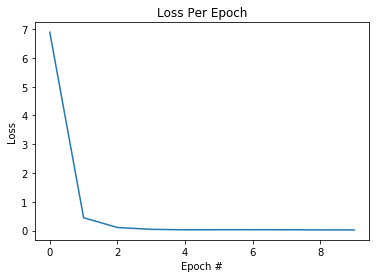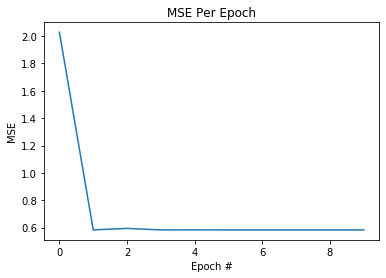<Figure size 432x288 with 0 Axes>

The plots above show that the model overfit to the training data. The training loss decreased to 0, while the MSE on the test set plateaued. Unsurprisingly, the neural network model predicted 0 for all test samples. Due to the small sample size, the network is unable to precisely regress the correct output value. Throwing more data at this model will likely help it generalize better since the upvote ratio is a continuous variable.

In :
modelPerformance = []

for i, output in enumerate(zip(testOutput, predictedRatio)):
target, prediction = output
modelPerformance.append({"Example" : i, "True Ratio" : target , "Predicted Ratio" : prediction})

modelPerformance = pd.DataFrame(modelPerformance)

Out:
Example True Ratio Predicted Ratio
0 0 0.95 0
1 1 0.50 0
2 2 0.49 0
3 3 0.52 0
4 4 0.87 0
In :
trainInput, testInput, trainOutput, testOutput = train_test_split(inputClassificationData, outputClassificationData, test_size=0.1)
testOutput = [i for i in testOutput]

class PredictRedditPost(nn.Module):
def __init__(self):
super(PredictRedditPost, self).__init__()
self.linearClassifier = nn.Sequential(nn.Linear(353, 64), nn.Dropout(0.5), nn.ReLU(),
nn.Linear(64, 16), nn.ReLU(),
nn.Linear(16, 1))
self.sigmoid = nn.Sigmoid()
#Same neural network architecture as above, now new and improved with a sigmoid activation function
def forward(self, featureVector):
return self.sigmoid(self.linearClassifier(featureVector))

In :
LR = 1e-3 #Learning Rate
WEIGHTDECAY=0.0005 #L2 Penalty which forces smaller weights and simpler models.
EPOCH = 10 #Number of iterations through the entire dataset

device = torch.device("cuda:0" if torch.cuda.is_available() else "cpu")
model = PredictRedditPost()
model.to(device)
BCEL = nn.BCELoss() #Binary Cross Entropy Loss used for two-class classifiation tasks

#Same training loop as regression case
TestingAccuracy = []
TrainingLoss = []
for STEP in range(1, EPOCH + 1):
epochLoss = 0
model.train()

for batchCount, data in enumerate(zip(trainInput, trainOutput)):
handCraftedFeatures, postType = data

handCraftedFeatures = torch.tensor(handCraftedFeatures) #Formatting features to play nice with PyTorch
postType = torch.tensor(postType)

handCraftedFeatures = handCraftedFeatures.to(device) #Send to GPU
postType = postType.to(device)

predictedPostType = model(handCraftedFeatures)

Loss = BCEL(predictedPostType, postType)
epochLoss = epochLoss + Loss.item()

Loss.backward()
optimizer.step()

print("Epoch " + str(STEP) + " Loss: " + str(epochLoss / batchCount))
TrainingLoss.append(epochLoss / batchCount)

model.eval()
testInput = torch.tensor(testInput)
testInput = testInput.to(device)
predictedPostType = torch.round(model(testInput))

predictedPostType = [int(i) for i in predictedPostType.tolist()]
Accuracy = metrics.accuracy_score(testOutput, predictedPostType)
print("Epoch "+ str(STEP) + " Accuracy: " + str(Accuracy) + "\n")
TestingAccuracy.append(Accuracy)

Epoch 1 Loss: 0.7118885397991939
Epoch 1 Accuracy: 0.7415730337078652

Epoch 2 Loss: 0.6699189038024655
Epoch 2 Accuracy: 0.7415730337078652

Epoch 3 Loss: 0.5273262527662723
Epoch 3 Accuracy: 0.7415730337078652

Epoch 4 Loss: 0.5522850346295222
Epoch 4 Accuracy: 0.848314606741573

Epoch 5 Loss: 0.4636425507706569
Epoch 5 Accuracy: 0.8876404494382022

Epoch 6 Loss: 0.45011415637895086
Epoch 6 Accuracy: 0.8595505617977528

Epoch 7 Loss: 0.4067802755341643
Epoch 7 Accuracy: 0.9438202247191011

Epoch 8 Loss: 0.45339107194554745
Epoch 8 Accuracy: 0.8539325842696629

Epoch 9 Loss: 0.40663455717023383
Epoch 9 Accuracy: 0.949438202247191

Epoch 10 Loss: 0.35305965436353764
Epoch 10 Accuracy: 0.9438202247191011


In :
sns.lineplot(x=np.array([x for x in range(EPOCH)]), y=np.array(TrainingLoss)) #Plot training loss per epoch
plt.title("Loss Per Epoch")
plt.xlabel("Epoch #")
plt.ylabel("Loss")
plt.show()
plt.clf()

sns.lineplot(x=np.array([x for x in range(EPOCH)]), y=np.array(TestingAccuracy)) #Plot classification accuracy per epoch
plt.title("Accuracy Per Epoch")
plt.xlabel("Epoch #")
plt.ylabel("Accuracy")
plt.show()
plt.clf()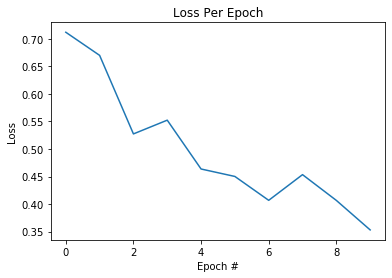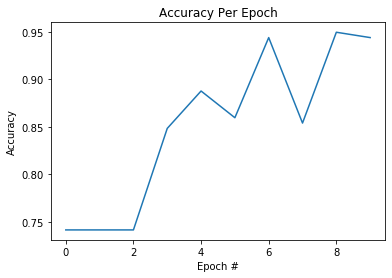<Figure size 432x288 with 0 Axes>

Our classification neural network is able to outperform our simple logistic regression model by a few percent. This state-of-the-art result should be published in next year's NeurIPS conference! Despite the apparent performance increase, neural networks are not interpretable. For the sake of explainability, it makes more sense to analyze the linear models.

The key takeaway from these experiments is that although many state-of-the-art methods use neural networks and deep learning, the size of the dataset plays an important role in whether to stick with traditional machine learning based approaches, or try deep learning models. Let's try to understand our features to see what really goes into making a great post. We'll be generating a Pearson Correlation Matrix to visualize how closely different features are correlated. For more information, read here. Here are the main takeaways:

1. Two variables have a significant correlation if their coefficient is at least 0.5
2. A variable is "important" for our model if it has a significant correlation with our label (in our case, upvote ratio, which is "UR" in the last column

As an important note, the limitations of the correlation function mean that we can only use numerical variables for this heatmap. That means we'll be omitting a categorical feature, subreddit, which we already know to be significant.

In :
numericalFeatureSelection = []

# Get numerical and boolean features, and abbreviate their labels
for tableRow in dataSet.iterrows():
lenTitle = tableRow["len_title"]
titleSentiment = tableRow["title_sentiment"]
titleSubjectivity = tableRow["title_subjectivity"]
titleQuestion  =tableRow["title_question"]
originalContent = tableRow["is_oc"]
nsfw = tableRow["is_nsfw"]
spoiler = tableRow["is_spoiler"]
lenBody = tableRow["len_body"]
post_type = tableRow["post_type"]
upvote_ratio = tableRow["upvote_ratio"]

numericalFeatureSelection.append({"TQ": titleQuestion, "LT" : lenTitle, "TSEN" : titleSentiment, "TSUB" : titleSubjectivity,
"OC" : originalContent, "NSFW" : nsfw, "SP" : spoiler,
"LB" : lenBody, "PT" : post_type, "UR" : upvote_ratio})

featureSelection = pd.DataFrame(numericalFeatureSelection)
plt.figure(figsize=(16,10))
pearsonCorrelation = featureSelection.corr() # Pearson's correlation coeff.
sns.heatmap(pearsonCorrelation, annot=True, cmap=plt.cm.Reds) # Using Seaborn to generate a heatmap
plt.show()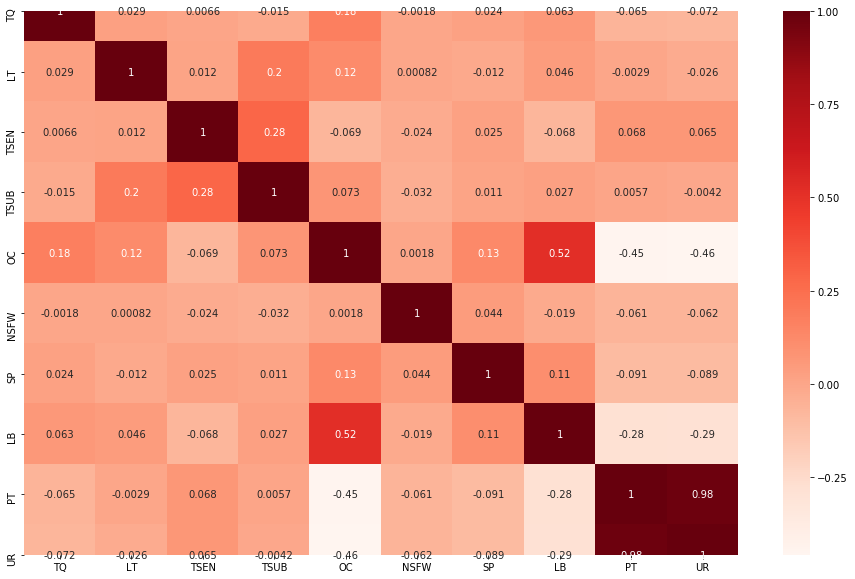In :
#Look Up Table for Above Plot
pprint({"TQ": "Title Contains Question","LT" : "Length of Tilte", "TSEN" : "Title Sentiment", "TSUB" : "Title Subjectivity",
"OC" : "Original Content", "NSFW" : "Not Safe for Work", "SP" : "Spoiler",
"LB" : "Length of Body", "PT" : "Post Type", "UR" : "Upvote Ratio"})

{'LB': 'Length of Body',
'LT': 'Length of Tilte',
'NSFW': 'Not Safe for Work',
'OC': 'Original Content',
'PT': 'Post Type',
'SP': 'Spoiler',
'TQ': 'Title Contains Question',
'TSEN': 'Title Sentiment',
'TSUB': 'Title Subjectivity',
'UR': 'Upvote Ratio'}


Throughout this tutorial, we have been trying to predict if a post will join the ranks of other top posts, or be condemned as a controversial post. The correlation matrix above shows that most numerical features of our dataset didn't actually affect the results of our linear models. Interestingly, both the length post body and the use of original content negatively correlated with both the post type (PT) and upvote ratio (UR). In order to have a hot post, make sure to always repost material and never write well-thought-out content!

Using this information, we can test our logistic regression model using only len_body and is_oc to predict post_type to see if we can get the same level of performance:

In :
inputClassificationData = []
outputClassificationData = []

for tableRow in dataSet.iterrows():
originalContent = tableRow["is_oc"]
lenBody = tableRow["len_body"]
post_type = tableRow["post_type"]

inputClassificationData.append([lenBody, originalContent])
outputClassificationData.append([post_type])

trainInput, testInput, trainOutput, testOutput = train_test_split(inputClassificationData, outputClassificationData, test_size=0.1)
ratioRegression = LogisticRegression().fit(np.array(trainInput), np.array(trainOutput))
predictedOutput = ratioRegression.predict(np.array(testInput))
modelPerformance = []

testOutput = [i for i in testOutput]
predictedOutput = predictedOutput.tolist()

Accuracy = metrics.accuracy_score(testOutput, predictedOutput)
print("Accuracy: " + str(Accuracy))

Accuracy: 0.7303370786516854


Although our logistic regression predictor is able to acheive "better than random" accuracy, the performance is still much lower than when we included other features. A noteable feature that we are missing is the subreddit itself.

In :
inputClassificationData = []
outputClassificationData = []

for tableRow in dataSet.iterrows():
subReddit = tableRow["subreddit"]
SR_oneHotEncoding = subRedditOneHotEncoding["subReddit_" + subReddit].to_list()
originalContent = tableRow["is_oc"]
lenBody = tableRow["len_body"]
post_type = tableRow["post_type"]

inputClassificationData.append(SR_oneHotEncoding + [lenBody, originalContent])
outputClassificationData.append([post_type])

trainInput, testInput, trainOutput, testOutput = train_test_split(inputClassificationData, outputClassificationData, test_size=0.1)
ratioRegression = LogisticRegression().fit(np.array(trainInput), np.array(trainOutput))
predictedOutput = ratioRegression.predict(np.array(testInput))
modelPerformance = []

testOutput = [i for i in testOutput]
predictedOutput = predictedOutput.tolist()

Accuracy = metrics.accuracy_score(testOutput, predictedOutput)
print("Accuracy: " + str(Accuracy))

Accuracy: 0.9101123595505618


With the subreddit included, our accuracy jumps from 73% to 91%! As suspected, the subreddit that you post in significantly impacts the likelihood of going viral on Reddit.

## Conclusion

To review, we scraped thousands of Reddit's best and worst posts, processed their features, performed some exploratory subreddit analysis, and attempted to predict a new post's performance with four different machine learning models. In the end, we have a working logistic regression model that can predict whether a post is more likely to be "top" or "controversial" with over 90% accuracy! If you'd like to make your own viral Reddit post, here are some of our suggestions:

1. Be persistent. Reddit has over 20 thousand daily submissions across all subreddits; it may take a few posts before people catch on to your content.
2. Post on a large, generic-topic subreddit. The ones that most frequently broke the top 1000 posts were r/pics, r/aww, r/funny, r/gifs, and r/gaming.
3. Post a link to an image or a video instead of original content. Posts with text had a significant negative correlation with upvote_ratio.
If you're interested in Reddit, there's so much data left to explore; the site hosts over 50 thousand subreddits and over 330 million users, so we've only touched on a small subset of the data that the site generates. Even if you're not interested, we hope this tutorial gave you some insight into how a hugely popular social news site chooses its favorite (and least favorite) posts. All in all, you're ready to go viral, and we hope it was worth the read!